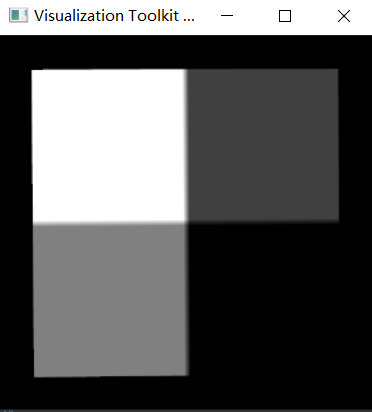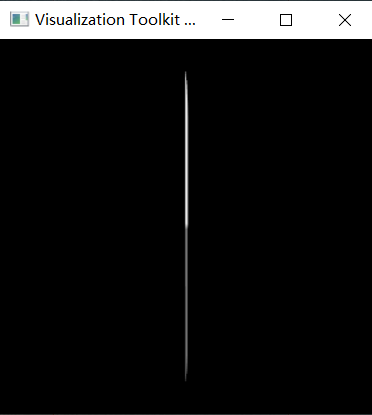# Does vtkRenderer consider the direction of vtkImageData?

I want to show a image with `vtkImageActor`, and there is no other prop. I hope the image is shown in the center of screen, so I apply `ResetCamera`. However, the shown image is strange:But the image should be:And the code to reproduce the result is:

``````import vtkmodules.all as vtk
import numpy as np
from BaseModule import numpyToVTK

img = np.zeros(shape=[50, 50])
img[25:, 0:25] = 64
img[0:25, 25:] = 128
img[25:, 25:] = 255
vtkImg = numpyToVTK(img)
vtkImg.SetOrigin(0, 0, 10)

dx = [0, 1, 0]
dy = [0, 0, 1]
dz = [1, 0, 0]
axialElement = [
dx, dy, dz,
dx, dy, dz,
dx, dy, dz
]
vtkImg.SetDirectionMatrix(axialElement)
imgActor = vtk.vtkImageActor()
imgActor.SetInputData(vtkImg)
imgActor.Update()

render = vtk.vtkRenderer()

renWin = vtk.vtkRenderWindow()
renWin.Render()
render.ResetCamera()

print('prop bounds: ', imgActor.GetBounds())
print('image bounds: ', vtkImg.GetBounds())

focalPoint = render.GetActiveCamera().GetFocalPoint()
position = render.GetActiveCamera().GetPosition()
print('active camera focal point is: ', focalPoint)
print('active camera position is: ', position)
print('image center is: ', vtkImg.GetCenter())

iren = vtk.vtkRenderWindowInteractor()
iren.SetRenderWindow(renWin)
iren.SetInteractorStyle(vtk.vtkInteractorStyleTrackballCamera())
iren.Initialize()
iren.Start()
``````

The printed information is:

``````prop bounds:  (0.0, 49.0, 0.0, 49.0, 10.0, 10.0)
image bounds:  (0.0, 0.0, 0.0, 49.0, 10.0, 59.0)
active camera focal point is:  (24.5, 24.5, 10.0)
active camera position is:  (24.5, 24.5, 143.870489570875)
image center is:  (0.0, 24.5, 34.5)
``````

We can see that the focal point of camera is not the center of vtkImageData.

Then, I read the source code of `ResetCamera`. It firstly compute the bounds of all props by `this->ComputeVisiblePropBounds(allBounds);`, and then set the focal point of camera as the center of bounds.

Unfortunately, the `vtkImg.GetBounds()` is not the same as `imgActor.GetBounds()`, because the `vtkImageData.GetBounds()` do not take the direction of `vtkImageData` into consideration when calculating the bounds.

Is my understanding correct? Is it a bug of vtk?

It looks to me that `vtkImageData.GetBounds()` takes Direction into account, but `vtkImageActor.GetBounds()` does not. That indicates a bug in `vtkImageActor`.

Interestingly, `vtkImageSlice` and `vtkImageSliceMapper`, which can be used instead of `vtkImageActor`, appear to do the Bounds correctly (at least, based on my inspection of the code).

Can you try the following code instead of `vtkImageActor`?

``````sliceMapper = vtk.vtkImageSliceMapper()
sliceMapper.SetInputData(vtkImg)

sliceActor = vtk.vtkImageSlice()
sliceActor.SetMapper(sliceMapper)

``````

After using `vtkImageSlice`, the output information is:

``````prop bounds:  (0.0, 0.0, 0.0, 49.0, 10.0, 59.0)
image bounds:  (0.0, 0.0, 0.0, 49.0, 10.0, 59.0)
active camera focal point is:  (0.0, 24.5, 34.5)
active camera position is:  (0.0, 24.5, 168.370489570875)
image center is:  (0.0, 24.5, 34.5)
``````

It is correct.

But, the display figure is:Which is still incorrect.

You rotated the image, but you didn’t rotate the camera. Try `camera.Azimuth(90.0)` or `camera.Azimuth(-90.0)` to rotate the camera by 90 degrees so that the camera faces the slice.

I’ll add some clarification. By default, the VTK camera points along the z direction. Calling ResetCamera() adjusts the Position and the FocalPoint, but never the direction. The direction of the camera must be changed manually.

@dgobbi I find another stranger thing.

After using `vtkImageSliceMapper` and `vtkImageSlice`, the bounds of actor is the same as that of vtkImg.

Then, I want to add `vtkImageMapToWindowLevelColors` in my pipeline as following:

``````vtkImg.SetDirectionMatrix(axialElement)
windowLevel = vtk.vtkImageMapToWindowLevelColors()
windowLevel.SetInputData(vtkImg)
windowLevel.Update()
sliceMapper = vtk.vtkImageSliceMapper()
# sliceMapper.SetInputData(vtkImg)
sliceMapper.SetInputConnection(windowLevel.GetOutputPort())
sliceMapper.Update()
sliceActor = vtk.vtkImageSlice()
sliceActor.SetMapper(sliceMapper)
``````

Then, the output information is different:

``````prop bounds:  (0.0, 49.0, 0.0, 49.0, 10.0, 10.0)
image bounds:  (0.0, 0.0, 0.0, 49.0, 10.0, 59.0)
``````

The bounds of vtkImg and actor is different.

Thus, the `vtkImageMapToWindowLevelColors` would change the bounds of mapper?

How to fix this bug?

Maybe I should using the vtkImageData which do not including direction information ~~~

I had to take some time to confirm it for myself, but you are absolutely correct that vtkImageMapToWindowLevelColors does not pass the Direction to its output. I looked through the code for vtkImageAlgorithm (which is the base class of all VTK image filters), and it completely ignores the Direction. This means that nearly all image filters will ignore the Direction.

In my own applications, my preference is to just use Spacing for the images (no Direction or Origin). Then I account for orientation and position by setting the orientation and position of the actor (i.e. the vtkImageSlice or vtkImageActor) that is displaying the data.

1 Like

@dgobbi Thank you for your reply. I will remove the direction information from `vtkImageData` in my project.

Hope vtk could fix this bug.

@dgobbi Sorry to bother you again. I find that this bug still is not be fixed for vtk 9.1.0.

Could you please tell me how to set the orientation for `vtkImageSlice` by `x=[x1, x2, x3], y=[y1, y2, y3]`. Thank you very much~~~

If the image orientation is `x=[x1, x2, x3], y=[y1, y2, y3], z=[z1, z2, z3]`, the code to display the image can be:

``````
sliceMapper = vtk.vtkImageSliceMapper()
sliceMapper.SetInputData(vtkImg)
imgActor = vtk.vtkImageSlice()
imgActor.SetMapper(sliceMapper)
imgActor.SetUserMatrix(matrix)

userMatrix = [
x1, y1, z1, pos1,
x2, y2, z2, pos2,
x3, y3, z3, pos3,
0, 0, 0, 1
]
matrix = vtk.vtkMatrix4x4()
matrix.DeepCopy(userMatrix)
imgActor.SetUserMatrix(matrix)
``````